Next: Methods Up: Introduction Previous: Maximum Likelihood

## Definitions

Definition 1.3   The optimal pairwise alignment OPA(s1, s2) of two sequences s1, s2 is an alignment with the maximum score where a probabilistic scoring method [5,14] is used. We refer to a pairwise alignment of two sequences s1, s2 with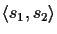.

Usually the optimal score is determined via standard dynamic programming [29,16]. An affine gap cost is used according to the formula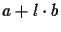, where a is a fixed gap cost, l is the length of the gap and b is the incremental cost .

Definition 1.4   A 1-PAM unit is the amount of evolution which will change, on average,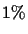of the amino acids. In mathematical terms, this is expressed as a matrix M such that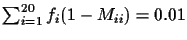, where fi is the frequency of the ith amino acid and Mij is the probability that an amino acid i mutates into an amino acid j at one PAM distance . The function PAM(s1, s2) is the PAM distance of two sequences si, s2 that maximizes the OPA score.

Definition 1.5   A Tree scoring function is a function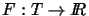.

Definition 1.6   Let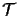be the set of all possible trees that can be generated for a given set of sequences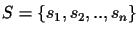. The optimal tree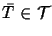is a tree such that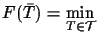F(T). (For some scoring functions we use the maximum instead of the minimum.)Next: Methods Up: Introduction Previous: Maximum Likelihood
Chantal Korostensky
1999-07-14